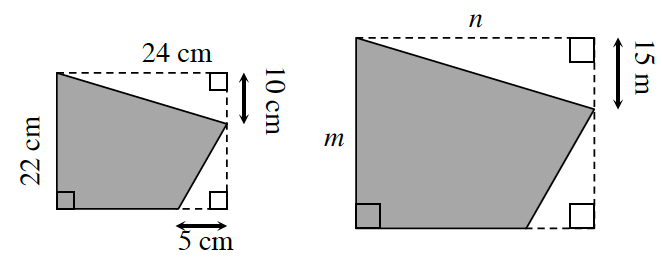### Home > CCG > Chapter Ch6 > Lesson 6.2.1 > Problem6-57

6-57.

The shaded figures below are similar. Homework Help ✎1. Solve for $m$ and $n$.

Use the proportion $\frac{22}{10}=\frac{m}{15}$ to find $m$.

Then write another portion for $n$.

2. Find the area and perimeter of each figure

Can you use the dotted lines to make right triangles so that you can solve for the lengths of the unknown sides?

Perimeter of smaller figure = $80$ cm

area of rectangle: $(24)(22)=528\text{ cm}^2$

area of top triangle: $\frac{1}{2}bh=\frac{1}{2}(10)(24)=120\text{ cm}^2$

area of bottom triangle: $\frac{1}{2}bh=\frac{1}{2}(5)(12)=30\text{ cm}^2$